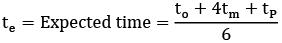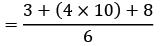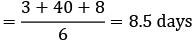Courses

# Test: CPM And PERT (Level - 1)

## 20 Questions MCQ Test Manufacturing and Industrial Engineering | Test: CPM And PERT (Level - 1)

Description
This mock test of Test: CPM And PERT (Level - 1) for Mechanical Engineering helps you for every Mechanical Engineering entrance exam. This contains 20 Multiple Choice Questions for Mechanical Engineering Test: CPM And PERT (Level - 1) (mcq) to study with solutions a complete question bank. The solved questions answers in this Test: CPM And PERT (Level - 1) quiz give you a good mix of easy questions and tough questions. Mechanical Engineering students definitely take this Test: CPM And PERT (Level - 1) exercise for a better result in the exam. You can find other Test: CPM And PERT (Level - 1) extra questions, long questions & short questions for Mechanical Engineering on EduRev as well by searching above.
QUESTION: 1

### In CPM network critical path denotes the

Solution: Total float in critical path is zero so delay in any activity will delay project.

QUESTION: 2

Solution:
QUESTION: 3

### A dummy activity

Solution:
QUESTION: 4

Dummy activities are used in a network to

Solution:
QUESTION: 5

Earliest finish time can be regarded as

Solution:
QUESTION: 6

In a network, total float is equal to?

Solution:
QUESTION: 7

A critical activity has

Solution:
QUESTION: 8

CPM has following time estimate

Solution:
QUESTION: 9

PERT has following time estimate

Solution:
QUESTION: 10

CPM is the

Solution:
QUESTION: 11

PERT is the

Solution:
QUESTION: 12

Consider the following statements PERT considers the following time estimates

Solution:
QUESTION: 13

The variance of the completion time for a project is the sum of variances of

Solution:
QUESTION: 14

The probability distribution of activity times in PERT follows following distribution

Solution:
QUESTION: 15

The probability distribution of project completion in PERT follows following distribution

Solution:
QUESTION: 16

If t o , t m and tP represent the optimistic, most probable and pessimistic time for a project, then by probability analysis, most probable expected time tE =

Solution:
QUESTION: 17

PERT stands for

Solution:
QUESTION: 18

The basic difference between PERT and CPM is that

Solution:
QUESTION: 19

In CPM, the cost slope is determined by

Solution:
QUESTION: 20

A PERT activity has an optimistic time estimate of 3 days, pessimistic time estimate of 8 days, and a most likely time estimate of 10 days. What is the expected time of this activity?

Solution: